# Characteristics of DC Motor | Shunt and Series Motor

Characteristics of dc motor play important role while selecting the motor for particular application. In General study, three characteristic curves are important for DC motors which are,

(i) Torque vs. armature current.

(ii) Speed vs. armature current.

(iii) Speed vs. torque.

These are explained below for DC shunt and DC Series Motor.

Question: Short note on Various Characteristic of a DC Motor:

Various characteristics of motor is to be used to judge performance of DC motor are as follows:

Torque – Armature current characteristics (T versus Ia)

• This is the characteristics which relates the torque and armature current.
• This characteristics is also called as the electrical characteristics of a motor.

Speed – Armature current characteristics (N versus Ia)

• This graph shows the relationship between speed and armature current.

Speed – Torque characteristics (N versus T)

• This is graph showing the relation between speed and torque.
• It is also called as the mechanical characteristics of a motor.

## Characteristics of DC Shunt Motor:

Question: Draw and explain the characteristics of dc shunt motor.

### Torque-Armature Current Characteristics:

This characteristics is plotted between armature current on x-axis and torque on y-axis as Shown in figure. We have stated that the torque (T) is directly proportional to the armature current (Ia) only since other parameters are constant.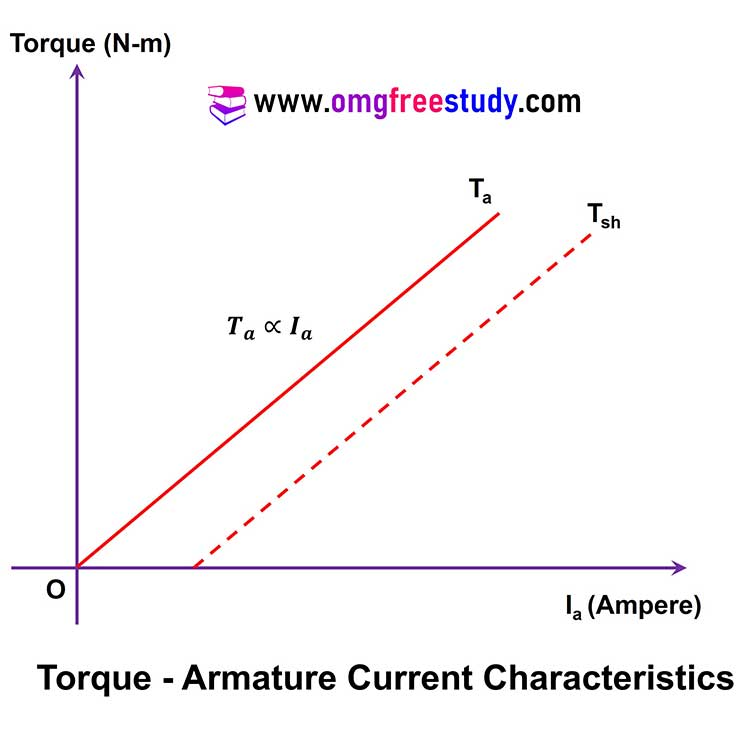This shows that the torque versus armature current characteristics is a straight line passing through the origin as shown in figure.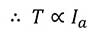As the load on the motor increases, armature current (Ia) increases proportionally and the torque (T) produced by the motor also increases linearly.

The torque (T) stated above is the gross torque (Ta) produced by the armature and k is proportionality constant.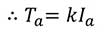### Speed-Armature Current Characteristics:

This is the graph of armature current (Ia) plotted on the X-axis and the corresponding speed (N) on the Y-axis.

The speed (in RPM) versus armature current characteristics of a DC shunt motor is shown in fig.  We know that the back emf is given by,From above equation pole (P), number of parallel path (A), Number of conductor (Z) are constants therefor we can write that.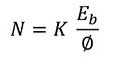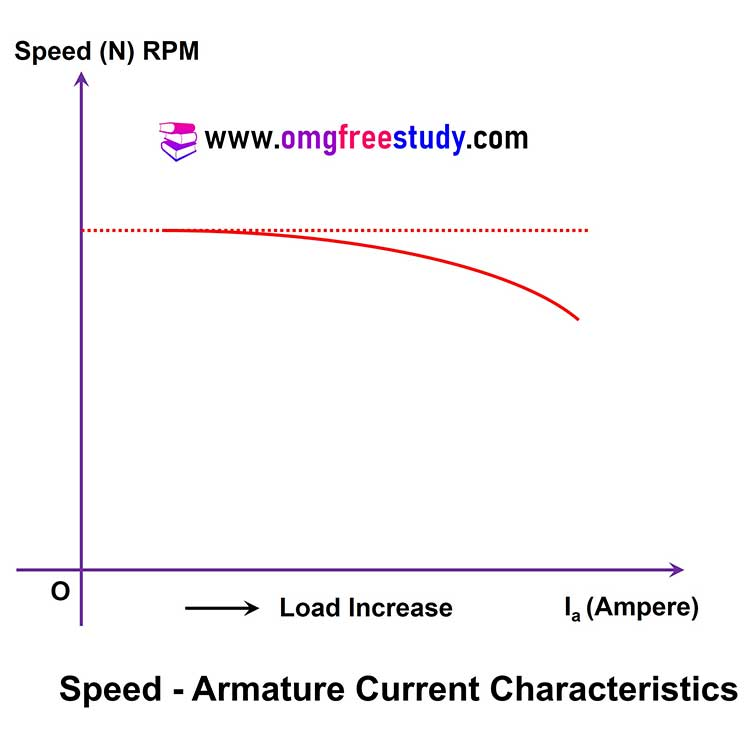For above equation speed (N) is inversely proportional to Flux  and Flux Φ is varies with current.

That’s mean we can conclude that if load on machine is increase current also increase and speed will decreases.

### Speed-Torque Characteristics:

The speed – torque characteristics of a dc motor is a graph of torque plotted on the X-axis versus the speed plotted on the Y-axis. The speed-torque characteristics of a DC shunt motor is as shown in figure.

As the torque is proportional to the armature current, the nature of this characteristics is same as that of the speed-armature current characteristics shown in fig.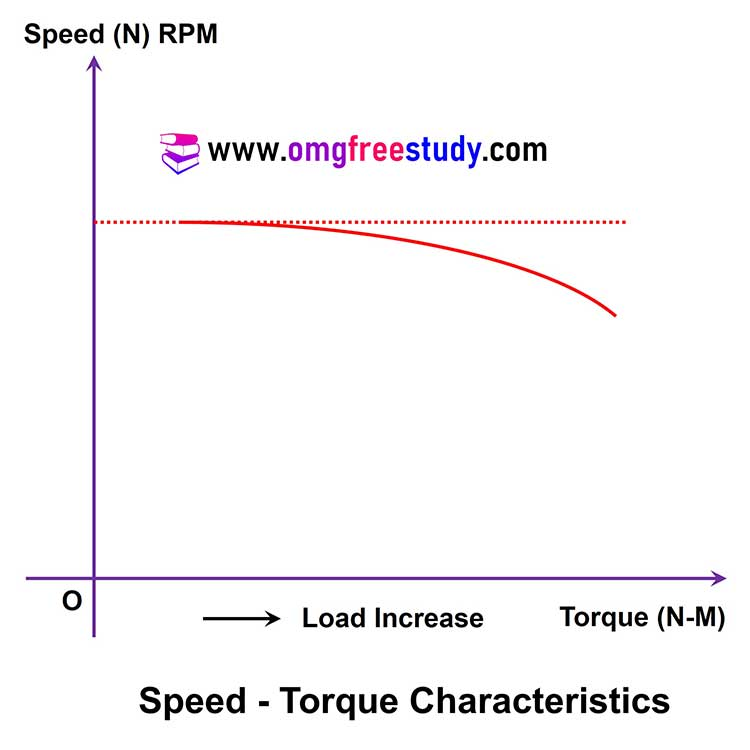To generate the required amount of torque, the motor has to draw more armature current and more armature current generate more flux therefor more flux reduce the speed of motor.

## Characteristics of DC Series Motor:

### Torque-Armature Current Characteristic:

The torque armature current characteristics of a DC series motor is as shown in fig.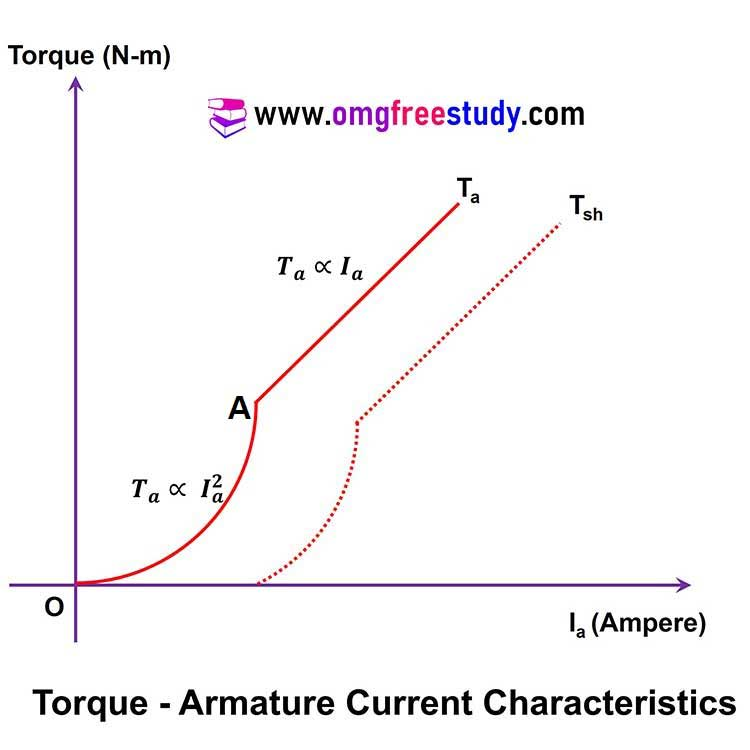For the DC series motor, the torque produced is given by,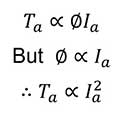Where Ta represents the gross torque produced by the motor.

As the torque is proportional to square of armature current, the starting torque of dc series motor is much higher than that of dc shunt motor.

The characteristics of Torque versus Armature can be divided into two parts:

In part-I, the torque is proportional to the square of armature current up to point ‘A’ in figure. Hence torque increases exponentially with increase in armature current.

In part-II of the characteristics in fig., the torque produced is directly proportional to the armature current.  Hence the torque increases linearly.

### Speed-Armature Current Characteristic:

The speed – armature current characteristics of a DC series motor is shown in figure.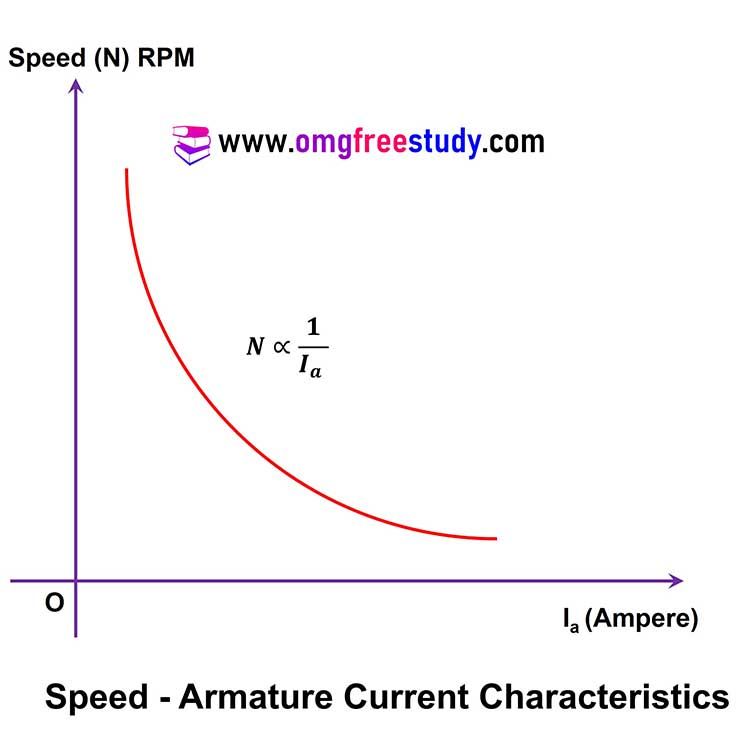We know that,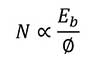The speed of motor is inversely proportional to the flux and flux is directly proportional to armature current, therefore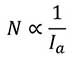Hence the speed of motor decreases with increases in armature current (Ia) as shown in fig.

### Speed-Torque Characteristic:

The speed – torque characteristics of a DC series motor is as shown in fig.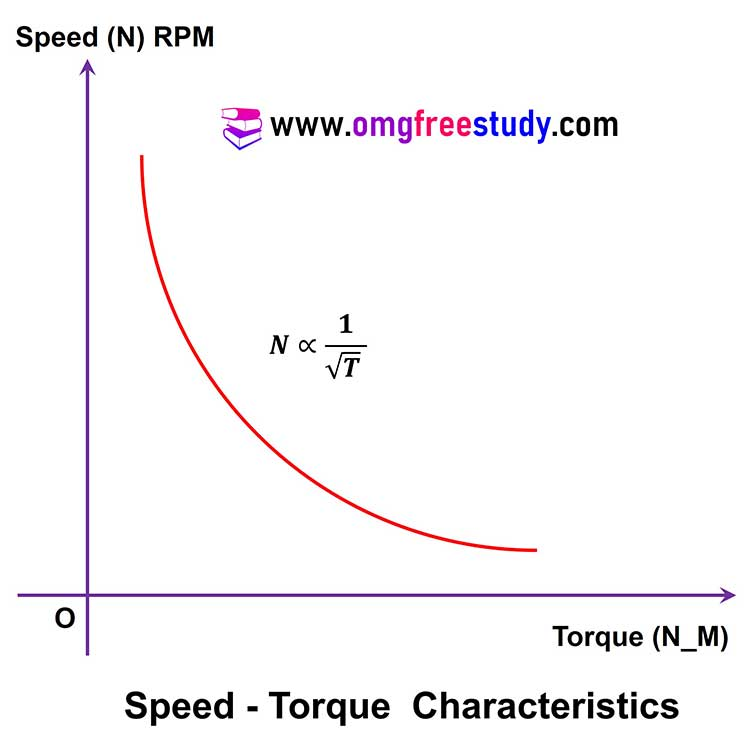We know that,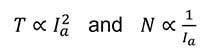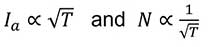This shows that the speed decreases with increase in the value of torque with increase in load.

Note that the nature of speed-torque characteristics is same as that of speed-armature current characteristics.

Watch MCQ Questions on DC motor And DC Generator on YouTube.

Scroll to Top
Scroll to Top